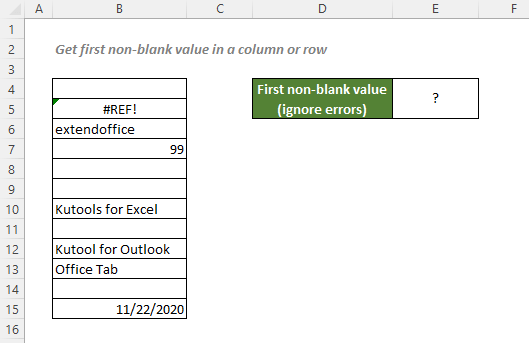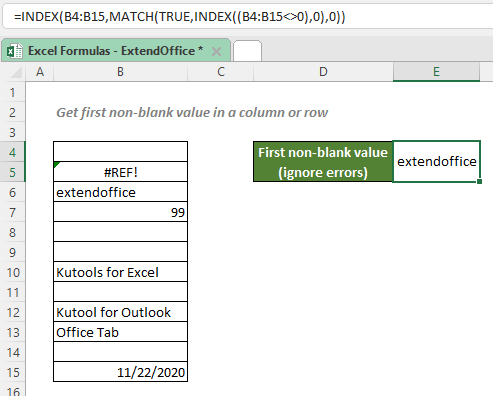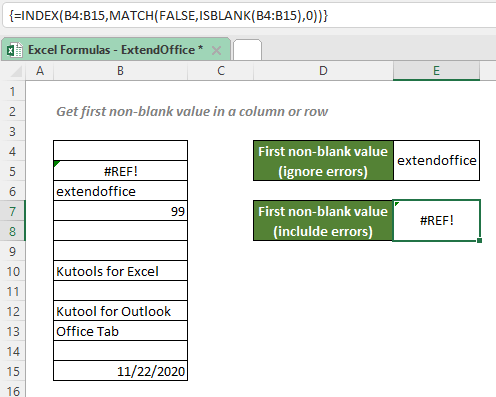Note: The other languages of the website are Google-translated. Back to English

## Get first non-blank value in a column or row

To retrieve the first value (the first cell that is not blank, ignoring errors) from a one-column or one-row range, you can use a formula based on INDEX and MATCH functions. However, if you don’t want to ignore the errors from your range, you can add an ISBLANK function to the above formula.#### Get first non-blank value in a column or row ignoring errors

To retrieve the first non-blank value in the list as shown above ignoring errors, you can use an INDEX function to find the cells that are not blank. Then you can use the MATCH to locate its position, and which will be fed to another INDEX to retrieve the value at the position.

#### Generic syntax

=INDEX(range,MATCH(TRUE,INDEX((range<>0),0),0))

• range: The one-column or one-row range where to return the first non-blank cell with text or number values while ignoring errors.

To retrieve the first non-blank value in the list ignoring errors, please copy or enter the formula below in the cell E4, and press Enter to get the result:

=INDEX(B4:B15,MATCH(TRUE,INDEX((B4:B15<>0),0),0))#### Explanation of the formula

=INDEX(B4:B15,MATCH(TRUE,INDEX((B4:B15<>0),0),0))

• INDEX((B4:B15<>0),0): The snippet evaluates each value in the range B4:B15. If a cell is blank, it will return a FLASE; If a cell contains an error, the snippet will return the error itself; And if a cell contains a number or text, a TRUE will be returned. Since the row_num argument of this INDEX formula is 0, so the snippet will return the array of values for the entire column like this: {FALSE;#REF!;TRUE;TRUE;FALSE;FALSE;TRUE;FALSE;TRUE;TRUE;FALSE;TRUE}.
• MATCH(TRUE,INDEX((B4:B15<>0),0),0) = MATCH(TRUE,{FALSE;#REF!;TRUE;TRUE;FALSE;FALSE;TRUE;FALSE;TRUE;TRUE;FALSE;TRUE},0): The match_type 0 forces the MATCH function to return the position of the first exact TRUE in the array. So, the function will return 3.
• INDEX(B4:B15,MATCH(TRUE,INDEX((B4:B15<>0),0),0)) = INDEX(B4:B15,3): The INDEX function then returns the 3rd value in the range B4:B15, which is extendoffice.

#### Get first non-blank value in a column or row including errors

To retrieve the first non-blank value in the list including errors, you can simply use the ISBLANK function to check the cells in the list if they are blank or not. Then INDEX will return the first non-blank value according to the position that MATCH provided.

#### Generic syntax

=INDEX(range,MATCH(FALSE,ISBLANK(range),0))

√ Note: This is an array formula that requires you to enter with Ctrl + Shift + Enter.

• range: The one-column or one-row range where to return the first non-blank cell with text, number or error values.

To retrieve the first non-blank value in the list including errors, please copy or enter the formula below in the cell E7, and press Ctrl + Shift + Enter to get the result:

=INDEX(B4:B15,MATCH(FALSE,ISBLANK(B4:B15),0))#### Explanation of the formula

=INDEX(B4:B15,MATCH(FALSE,ISBLANK(B4:B15),0))

• ISBLANK(B4:B15): The ISBLANK function checks if the cells in the range B4:B15 are blank or not. If yes, a TRUE will be returned; If not, a FALSE will be returned. So, the function will generate an array like this: {TRUE;FALSE;FALSE;FALSE;TRUE;TRUE;FALSE;TRUE;FALSE;FALSE;TRUE;FALSE}.
• MATCH(FALSE,ISBLANK(B4:B15),0) = MATCH(FALSE,{TRUE;FALSE;FALSE;FALSE;TRUE;TRUE;FALSE;TRUE;FALSE;FALSE;TRUE;FALSE},0): The match_type 0 forces the MATCH function to return the position of the first exact FALSE in the array. So, the function will return 2.
• INDEX(B4:B15,MATCH(FALSE,ISBLANK(B4:B15),0)) = INDEX(B4:B15,2): The INDEX function then returns the 2nd value in the range B4:B15, which is #REF!.

#### Related functions

Excel INDEX function

The Excel INDEX function returns the displayed value based on a given position from a range or an array.

Excel MATCH function

The Excel MATCH function searches for a specific value in a range of cells, and returns the relative position of the value.

#### Related Formulas

Exact match with INDEX and MATCH

If you need to find out the information listed in Excel about a specific product, movie or a person, etc., you should make a good use of the combination of INDEX and MATCH functions.

Get first text value in a column

To retrieve the first text value from a one-column range, you can use a formula based on INDEX and MATCH functions as well as a formula based on the VLOOKUP function.

Locate first partial match with wildcards

There are cases that you need to get the position of the first partial match that contains specific number in a range of numeric values in Excel. In this case, a MATCH and TEXT formula that incorporates asterisk (*), the wildcard that matches any number of characters, will do you a favor. And if you also need to know the exact value at that position, you can add the INDEX function to the formula.

Lookup the first partial match number

There are cases that you need to get the position of the first partial match that contains specific number in a range of numeric values in Excel. In this case, a MATCH and TEXT formula that incorporates asterisk (*), the wildcard that matches any number of characters, will do you a favor. And if you also need to know the exact value at that position, you can add the INDEX function to the formula.

### The Best Office Productivity Tools

#### Kutools for Excel - Helps You To Stand Out From Crowd

Would you like to complete your daily work quickly and perfectly? Kutools for Excel brings 300 powerful advanced features (Combine workbooks, sum by color, split cell contents, convert date, and so on...) and save 80% time for you.

• Designed for 1500 work scenarios, helps you solve 80% Excel problems.
• Reduce thousands of keyboard and mouse clicks every day, relieve your tired eyes and hands.
• Become an Excel expert in 3 minutes. No longer need to remember any painful formulas and VBA codes.
• 30-day unlimited free trial. 60-day money back guarantee. Free upgrade and support for 2 years.#### Office Tab - Enable Tabbed Reading and Editing in Microsoft Office (include Excel)

• One second to switch between dozens of open documents!
• Reduce hundreds of mouse clicks for you every day, say goodbye to mouse hand.
• Increases your productivity by 50% when viewing and editing multiple documents.
• Brings Efficient Tabs to Office (include Excel), Just Like Chrome, Firefox, And New Internet Explorer.